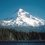Behold the Power of the Sun!Main post link -> http://blog.brilliant.org/2013/02/10/behold-the-power-of-the-sun/

Here is the solution to last week's problem of the week. For a recap of the problem check the blog or here. Around 90 people solved it correctly last week. This next weekend one of them will be announced as the winner of a free t-shirt. Feel free to discuss the problem it's solution. Did you solve it a different way?

Since we can ignore convective or conductive heat transfer, the only way the cube will lose energy due to radiation into the surrounding area. The power loss due to radiation is given by $P=A_c \sigma \epsilon(T^4-T_0^4)$, where $A_c$ is the surface area of the cube, $\sigma$ is the Stefan-Boltzmann constant, $\epsilon$ is the emissivity, T is the temperature of the cube and $T_0$ is the temperature of the air. Note that you cannot neglect the temperature of the air in this problem! Also, as a fun aside, the energy loss rate scaling as the temperature of the object to the fourth power is one of the reasons you feel 'cold' when you have a fever. Our body is actually sensitive to the rate of energy loss, so when you have a fever you lose energy more quickly and feel cold.

Returning to our problem, since the cube is a perfect blackbody, $\epsilon=1$ and we can then calculate a total power loss of $4083$ Watts. This loss must be compensated for by the energy coming in from the mirrors, and so the total area of the mirrors is 4.083 $m^2$.Note by Peter Taylor
6 years, 8 months ago

This discussion board is a place to discuss our Daily Challenges and the math and science related to those challenges. Explanations are more than just a solution — they should explain the steps and thinking strategies that you used to obtain the solution. Comments should further the discussion of math and science.

When posting on Brilliant:

• Use the emojis to react to an explanation, whether you're congratulating a job well done , or just really confused .
• Ask specific questions about the challenge or the steps in somebody's explanation. Well-posed questions can add a lot to the discussion, but posting "I don't understand!" doesn't help anyone.
• Try to contribute something new to the discussion, whether it is an extension, generalization or other idea related to the challenge.
• Stay on topic — we're all here to learn more about math and science, not to hear about your favorite get-rich-quick scheme or current world events.

MarkdownAppears as
*italics* or _italics_ italics
**bold** or __bold__ bold

- bulleted
- list

• bulleted
• list

1. numbered
2. list

1. numbered
2. list
Note: you must add a full line of space before and after lists for them to show up correctly
paragraph 1

paragraph 2

paragraph 1

paragraph 2

> This is a quote
This is a quote
# I indented these lines
# 4 spaces, and now they show
# up as a code block.

print "hello world"
# I indented these lines
# 4 spaces, and now they show
# up as a code block.

print "hello world"
MathAppears as
Remember to wrap math in $$...$$ or $...$ to ensure proper formatting.
2 \times 3 $2 \times 3$
2^{34} $2^{34}$
a_{i-1} $a_{i-1}$
\frac{2}{3} $\frac{2}{3}$
\sqrt{2} $\sqrt{2}$
\sum_{i=1}^3 $\sum_{i=1}^3$
\sin \theta $\sin \theta$
\boxed{123} $\boxed{123}$

Sort by:

What made no sense in this question, is the assumption that all the 6 sides of the collector are radiating as a black body, where only 4 sides(vertical areas) are used as collectors. The top and the bottom of the cube should not be made as black body, but on the contrary to prevent losses should be made of reflective material (perfect as possible) to contain the heat in the cube. So sometimes common sense will lead you to the wrong answer:)

- 6 years, 8 months ago

It was also my idea, in fact at first I considered only 4 sides. But eventually I asked and I was told to consider all 6 sides.

- 6 years, 8 months ago